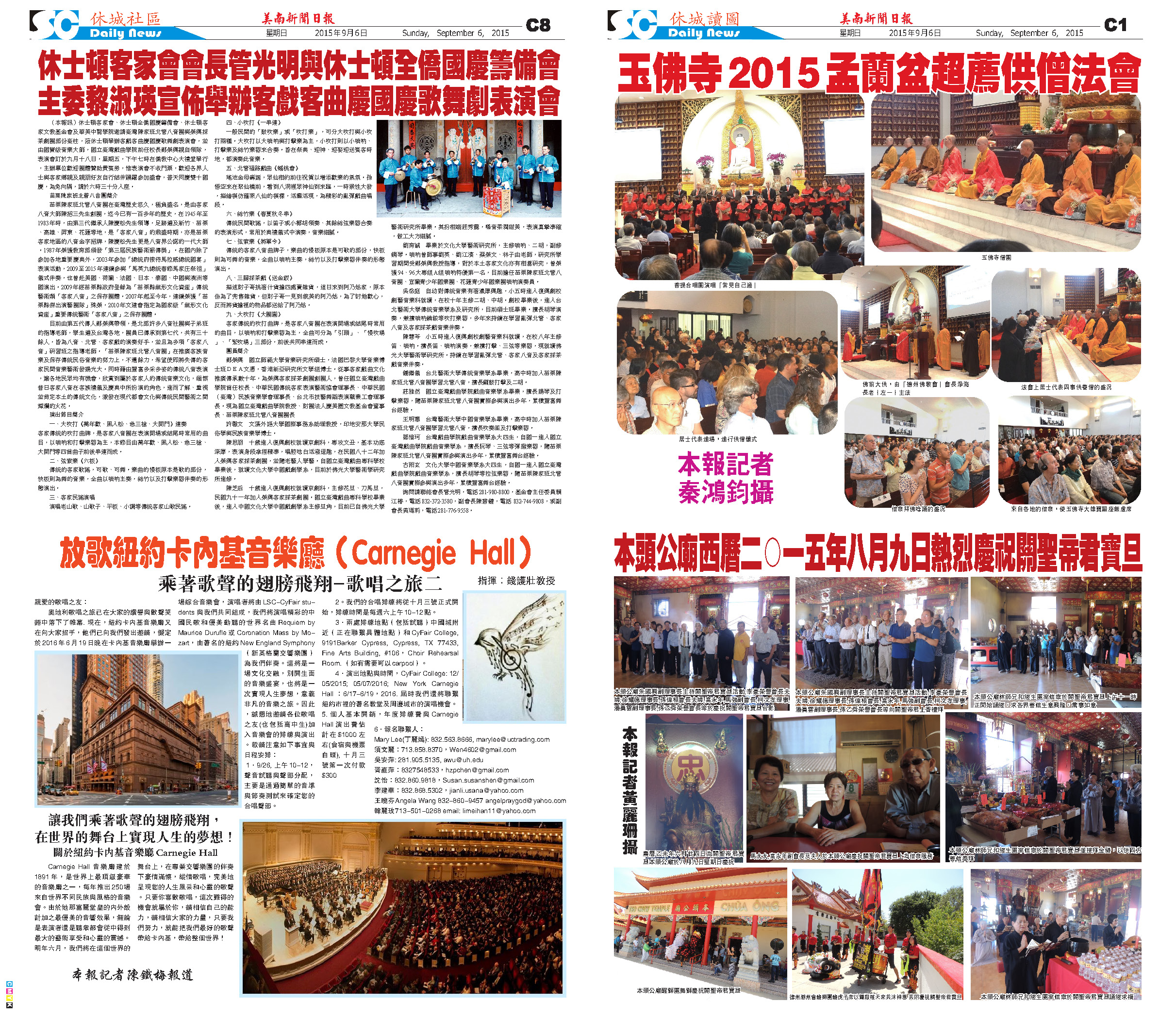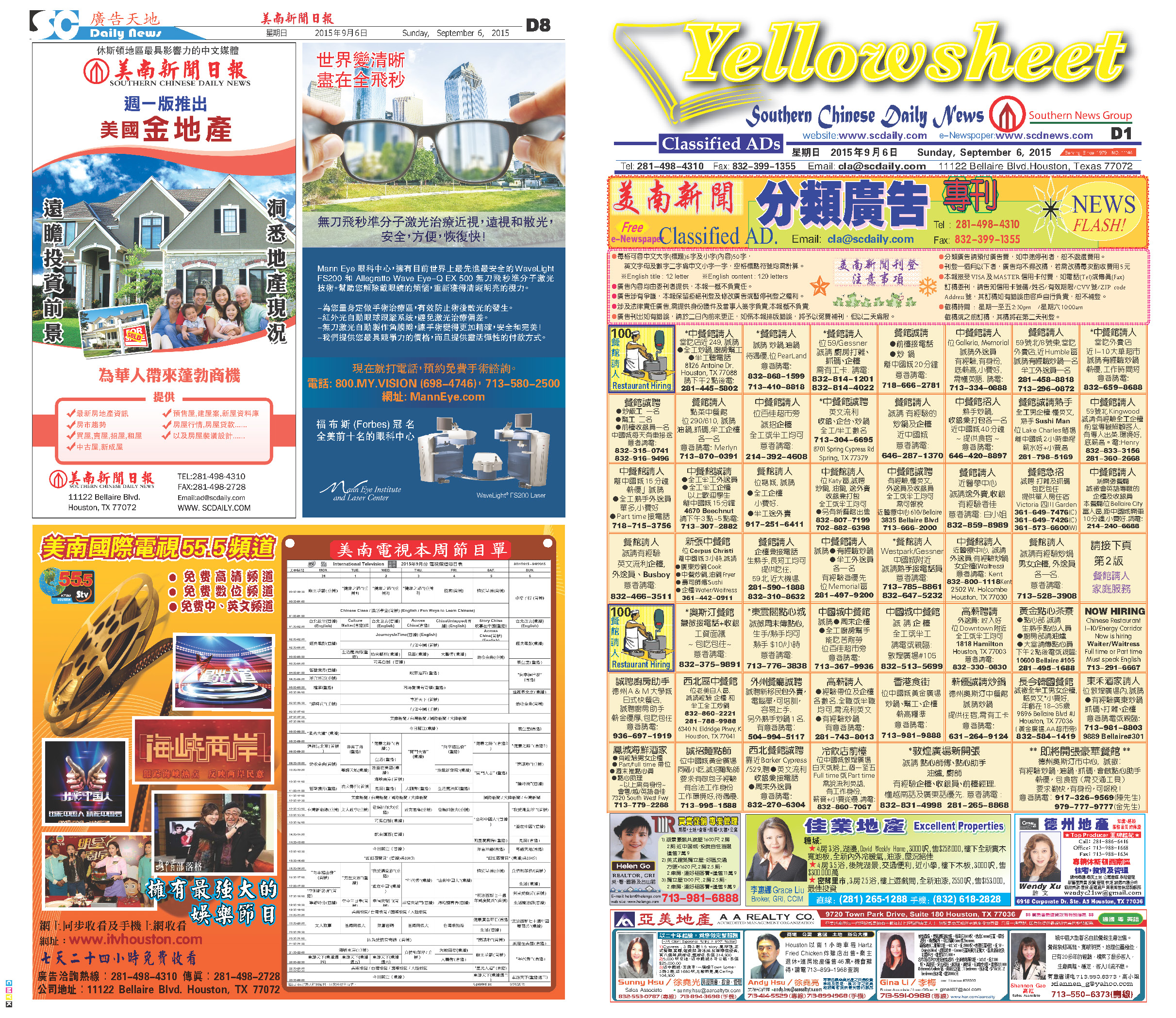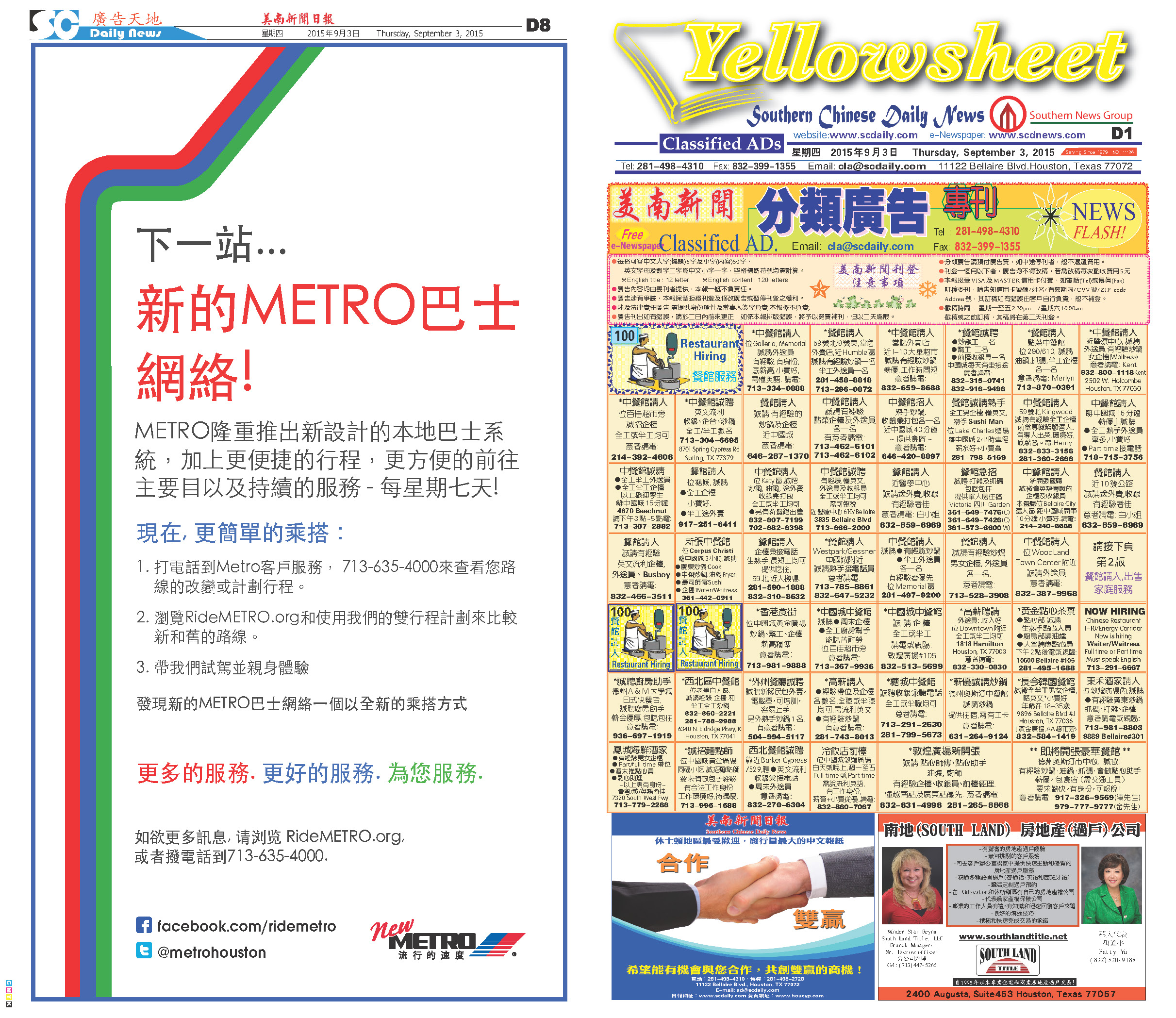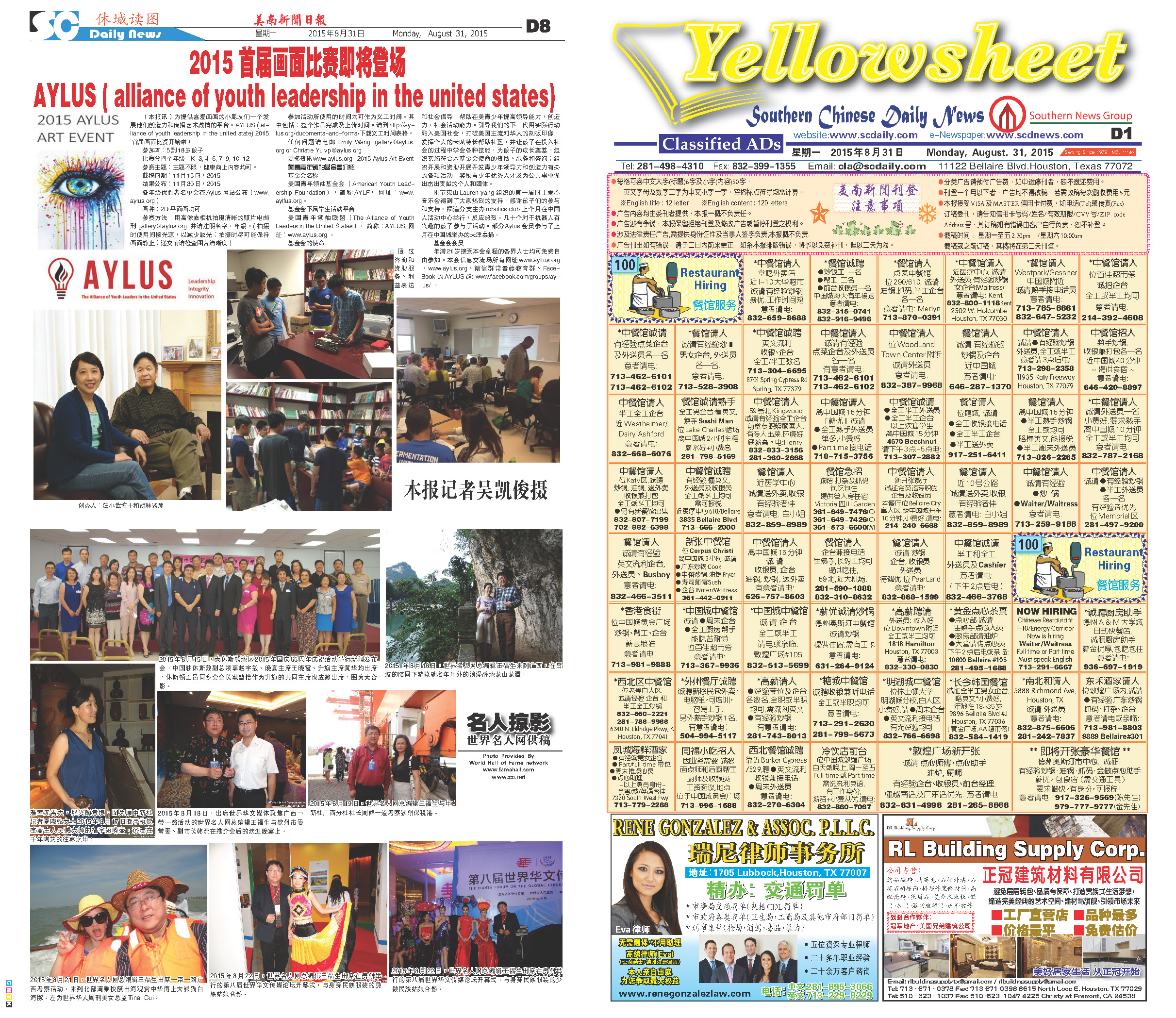150906 Epaper

 A Section B SectionC Section D Section150905 Epaper

 A Section B SectionC Section D Section150904 Epaper

 A Section B SectionC Section D Section150903 Epaper

 A Section B SectionC Section D Section150902 Epaper

 A Section B SectionC Section D Section150901 Epaper

 A Section B SectionC Section D Section150831 Epaper

 A Section B SectionC Section D Section150830 Epaper

 A Section B SectionC Section D Section150828 Epaper

 A Section B SectionC Section D Section150829 Epaper

 A Section B SectionC Section D Section150827 Epaper

 A Section B SectionC Section D Section